# Conditional Probability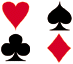Problem: A math teacher gave her class two tests. 25% of the class passed both tests and 42% of the class passed the first test. What percent of those who passed the first test also passed the second test?

Analysis: This problem describes a conditional probability since it asks us to find the probability that the second test was passed given that the first test was passed. In the last lesson, the notation for conditional probability was used in the statement of Multiplication Rule 2.

Multiplication Rule 2: When two events, A and B, are dependent, the probability of both occurring is:The formula for the Conditional Probability of an event can be derived from Multiplication Rule 2 as follows: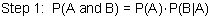Start with Multiplication Rule 2.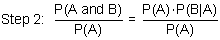Divide both sides of equation by P(A).Cancel P(A)s on right-hand side of equation.Commute the equation.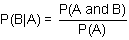We have derived the formula for conditional probability.

Now we can use this formula to solve the problem at the top of the page.

Problem: A math teacher gave her class two tests. 25% of the class passed both tests and 42% of the class passed the first test. What percent of those who passed the first test also passed the second test?

Solution:

 P(Second|First) = P(First and Second) = 0.25 = 0.60 = 60% P(First) 0.42

Let's look at some other problems in which we are asked to find a conditional probability.

Example 1: A jar contains black and white marbles. Two marbles are chosen without replacement. The probability of selecting a black marble and then a white marble is 0.34, and the probability of selecting a black marble on the first draw is 0.47. What is the probability of selecting a white marble on the second draw, given that the first marble drawn was black?

Solution:

 P(White|Black) = P(Black and White) = 0.34 = 0.72 = 72% P(Black) 0.47

Example 2: The probability that it is Friday and that a student is absent is 0.03. Since there are 5 school days in a week, the probability that it is Friday is 0.2. What is the probability that a student is absent given that today is Friday?

Solution:

 P(Absent|Friday) = P(Friday and Absent) = 0.03 = 0.15 = 15% P(Friday) 0.2

Example 3: At Kennedy Middle School, the probability that a student takes Technology and Spanish is 0.087. The probability that a student takes Technology is 0.68. What is the probability that a student takes Spanish given that the student is taking Technology?

Solution:

 P(Spanish|Technology) = P(Technology and Spanish) = 0.087 = 0.13 = 13% P(Technology) 0.68

Summary: The conditional probability of an event B in relationship to an event A is the probability that event B occurs given that event A has already occurred. The notation for conditional probability is P(B|A), read as the probability of B given A. The formula for conditional probability is:The Venn Diagram below illustrates P(A), P(B), and P(A and B). What two sections would have to be divided to find P(B|A)?   Answer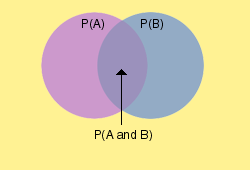### Exercises

Directions: Read each question below. Select your answer by clicking on its button. Feedback to your answer is provided in the RESULTS BOX. If you make a mistake, choose a different button. Answer choices have been rounded to the nearest percent.

 1. In New York State, 48% of all teenagers own a skateboard and 39% of all teenagers own a skateboard and roller blades. What is the probability that a teenager owns roller blades given that the teenager owns a skateboard? 87%81%123%None of the above. RESULTS BOX:
 2. At a middle school, 18% of all students play football and basketball and 32% of all students play football. What is the probability that a student plays basketball given that the student plays football? 56%178%50%None of the above. RESULTS BOX:
 3. In the United States, 56% of all children get an allowance and 41% of all children get an allowance and do household chores. What is the probability that a child does household chores given that the child gets an allowance? 137%97%73%None of the above. RESULTS BOX:
 4. In Europe, 88% of all households have a television. 51% of all households have a television and a VCR. What is the probability that a household has a VCR given that it has a television? 173%58%42%None of the above. RESULTS BOX:
 5. In New England, 84% of the houses have a garage and 65% of the houses have a garage and a back yard. What is the probability that a house has a backyard given that it has a garage? 77%109%19%None of the above. RESULTS BOX: